Vectors and conics

This free course is available to start right now. Review the full course description and key learning outcomes and create an account and enrol if you want a free statement of participation.

Free course

1.5 Distance between two points in the plane

Next, we find the formula for the distance between two points P (x1, y1) and Q(x2, y2) in the plane. In the diagram below we have drawn P and Q in the first quadrant, but the formula we derive holds wherever the points are in the plane.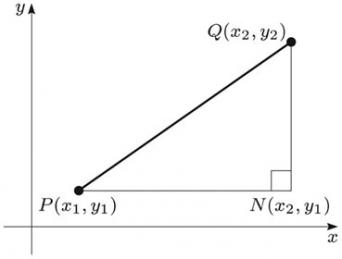We can construct a right-angled triangle PNQ as shown; the line PN is parallel to the x-axis, the line QN is parallel to the y-axis, the angle PNQ is a right angle, and PQ is the hypotenuse of the triangle.

The length of PN is |x2x1| and the length of QN is |y2y1|.

Note: For any real number x,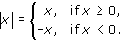It follows from Pythagoras' Theorem that PQ2 = PN2 + QN2, so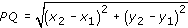Distance Formula in two-dimensional Euclidean space

The distance between two points (x1, y1) and (x2, y2) in the plane is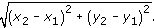For example, it follows from the formula above that the distance between the points (1, 2) and (3, −4) is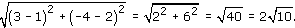Example 5

Find the distance between each of the following pairs of points in the plane.

• (a) (0, 0) and (5, 0).

• (b) (0, 0) and (3, 4).

• (c) (1, 2) and (5, 1).

• (d) (3, −8) and (−1, 4).

We use the formula for the distance between two points in the plane. This gives the following distances.

• (a)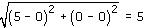• (b)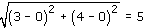• (c)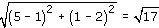• (d)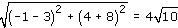M208_1